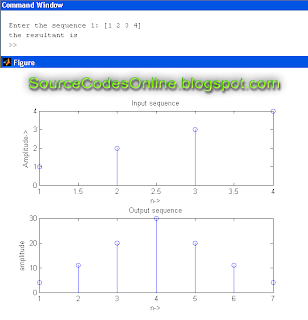# MATLAB Program for Auto Correlation for the given sequence | IT1254 - DSP and Communications Systems Lab

AIM:
To write a program for Auto Correlation for the given sequence using MATLAB in IT1254 - DSP and Communications Systems Lab.

ALGORITHM:
Step 1: Start
Step 2: Read the input sequence
Step 3: Perform auto correlation using the xcorr() function
Step 4: Plot the sequences
Step 5: Display the input & output sequences
Step 6: Stop

SOURCE CODE:
clc;
close all;
clear all;
x=input('Enter the sequence 1: ');
y=xcorr(x,x);
figure;
subplot(2,1,1);
stem(x);
ylabel('Amplitude->');
xlabel('n->');
title('Input sequence');
subplot(2,1,2);
stem(fliplr(y));
ylabel('amplitude');
xlabel('n->');
title('Output sequence');
disp('the resultant is ');
fliplr(y);
OUTPUT:Click to view full size image!

RESULT:
Thus the program for auto correlation of a given sequence was calculated and the output was displayed.
Previous
Next Post »